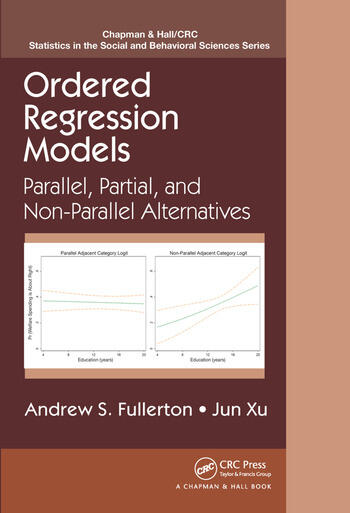Ordered Regression Models: Parallel, Partial, and Non-Parallel Alternatives

1st Edition

Andrew S. Fullerton, Jun Xu

Chapman and Hall/CRC
Published April 5, 2016
Reference - 172 Pages - 18 B/W Illustrations
ISBN 9781466569737 - CAT# K16454
Series: Chapman & Hall/CRC Statistics in the Social and Behavioral Sciences

For Instructors Request Inspection Copy

was \$85.95

USD\$68.76

SAVE ~\$17.19

FREE Standard Shipping!

Preview

Summary

Estimate and Interpret Results from Ordered Regression Models

Ordered Regression Models: Parallel, Partial, and Non-Parallel Alternatives presents regression models for ordinal outcomes, which are variables that have ordered categories but unknown spacing between the categories. The book provides comprehensive coverage of the three major classes of ordered regression models (cumulative, stage, and adjacent) as well as variations based on the application of the parallel regression assumption.

The authors first introduce the three "parallel" ordered regression models before covering unconstrained partial, constrained partial, and nonparallel models. They then review existing tests for the parallel regression assumption, propose new variations of several tests, and discuss important practical concerns related to tests of the parallel regression assumption. The book also describes extensions of ordered regression models, including heterogeneous choice models, multilevel ordered models, and the Bayesian approach to ordered regression models. Some chapters include brief examples using Stata and R.

This book offers a conceptual framework for understanding ordered regression models based on the probability of interest and the application of the parallel regression assumption. It demonstrates the usefulness of numerous modeling alternatives, showing you how to select the most appropriate model given the type of ordinal outcome and restrictiveness of the parallel assumption for each variable.

Web Resource
More detailed examples are available on a supplementary website. The site also contains JAGS, R, and Stata codes to estimate the models along with syntax to reproduce the results.

Instructors

We provide complimentary e-inspection copies of primary textbooks to instructors considering our books for course adoption.